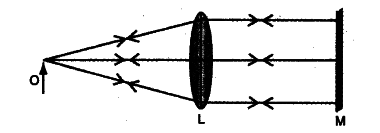# The ray diagram given below illustrates the experimental set up for the determination of the focal length of a converging lens using a plane mirror

The ray diagram given below illustrates the experimental set up for the determination of the focal length of a converging lens using a plane mirror.
(i) State the magnification of the image formed.
(ii) Write two characteristics of the image formed.
(iii) What is the name given to the distance between the object and optical centre of the lens in the given diagram ?(i) The magnification of the image formed is unity (or 1)
(ii) The image formed is (1) real and (2) inverted, is at the same position as the object.
(iii) The distance between the object and the optical centre of the lens, is called the focal length of the lens.